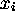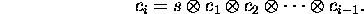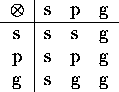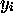# Parallel Prefix Computations

Tom Kelliher, CS 315

Mar. 3, 1999

### Outline

1. Introduction.

2. Computing the prefixes.

# Parallel Prefix Computations

## Introduction

1. General form of a prefix computation:whereis a function of an input bit.

2. Consider carry lookahead addition. Letbe the s, p, or g for bit position i. Then:Note that this is written ``backwards.''

Why the initial s?This operation must be associative for the carry computation to work. Why? Show.

What's going on here?

## Computing the Prefixes

1. Using a four-leaf tree, label each leaf with it'sandvalues.

2. Can the class derive the calculation for the tree? Hint: each vertex in the tree stores the product of its sub-tree leaves.

3. General idea:
1. Rising phase: each vertex computes the product of inputs from its children. Action of a leaf, internal vertex.

2. Computed values are passed from left child to right child.

3. Falling phase: each internal vertex takes received value and transmits it to children. Leaves concatenate received values on left.

4. Show an example on a nine-leaf tree.

Thomas P. Kelliher
Wed Mar 3 11:16:47 EST 1999
Tom Kelliher